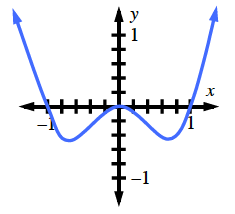### Home > APCALC > Chapter 3 > Lesson 3.2.1 > Problem3-43

3-43.

Write and evaluate a Riemann sum to estimate the area under the curve for $−1\le x\le1$ given $f(x) = x^4 − x^2$. Choose the number of rectangles so that your answer will be a good approximation of the area. What is interesting about the sign of your result? Use a description or sketch of the function to support your answer.Your answer should be relatively close to $\approx−0.26667$. The answer is negative because the function is below the $x$-axis on this interval.